# NCERT Solutions For Class 10 Maths Chapter 2 Polynomials

Here are the NCERT Solutions for Class 10 Maths Chapter 2 Polynomials to help students prepare for their exams. These solutions were written by Math topic experts to help students prepare for their first-term exams. They approach these issues in such a way that students can practice the questions from Chapter 2 Polynomials using the NCERT Solutions. Students will find it easy to understand these Maths NCERT Class 10 Solutions because they include step-by-step explanations.

NCERT Solutions for Class 10 Maths would be a great study resource for students. Students who complete these Polynomials NCERT Solutions for Class 10 Maths will perform well on their first and second semester exams. Special attention is made to following the new CBSE Syllabus for 2022-23 when generating these solutions.

This is one of the most important topics in math, hence it belongs under the Algebra unit of the Class 10 Maths CBSE Term I exams, which has a 20-mark weighted. One question is the typical number of questions posed from this chapter.

NCERT Solutions for Class 10 Maths Chapter 2- Polynomials- Free PDF Download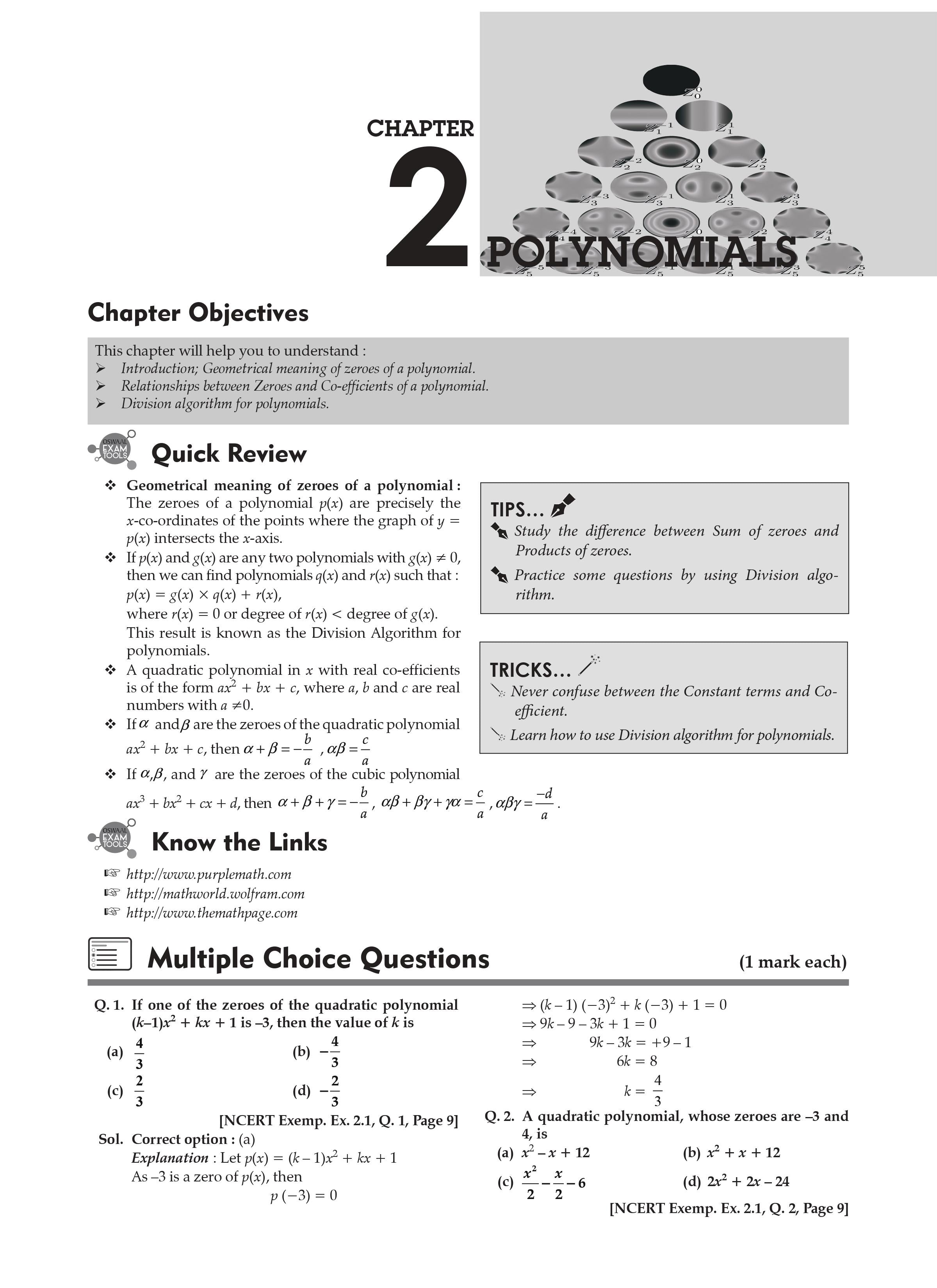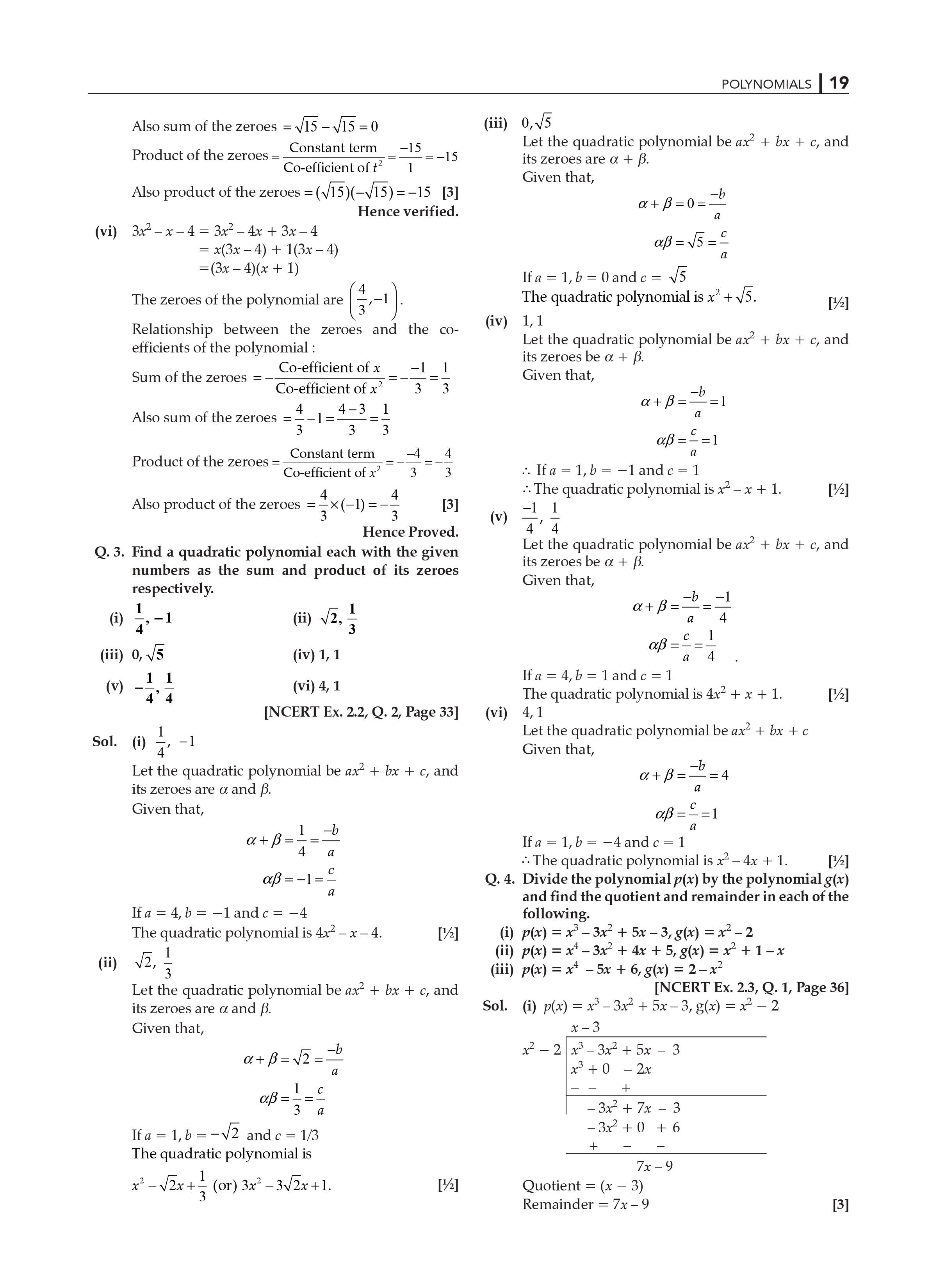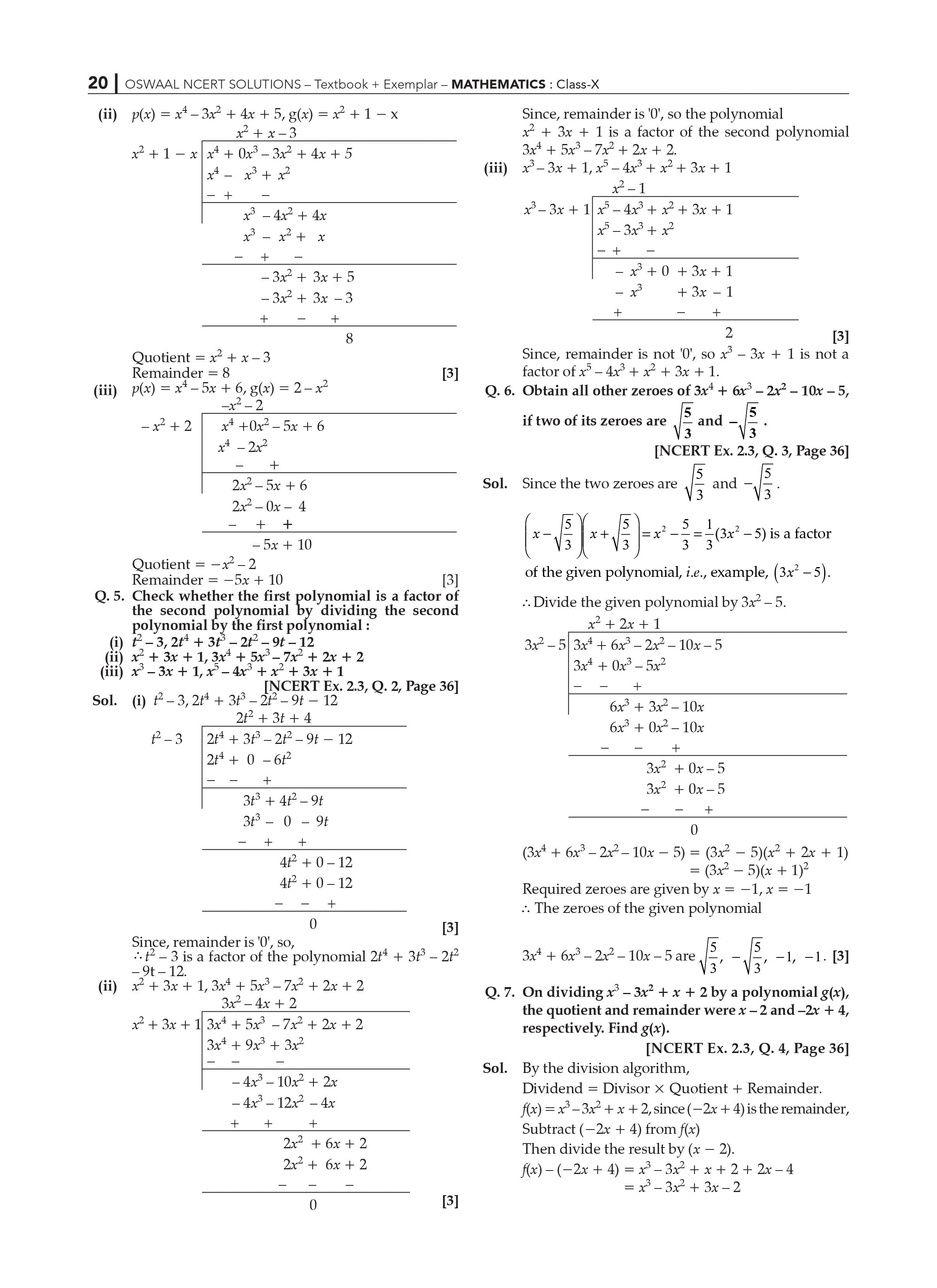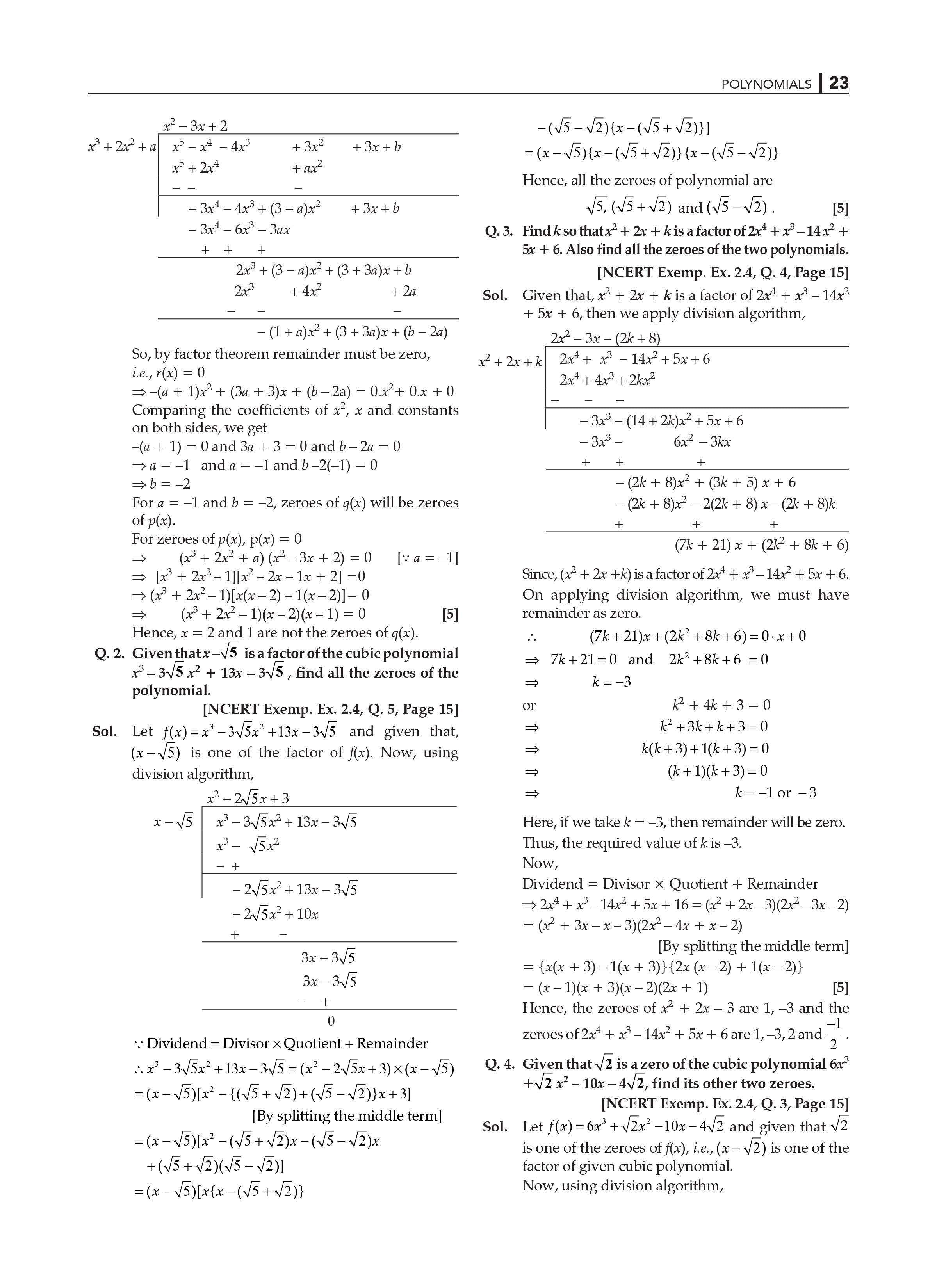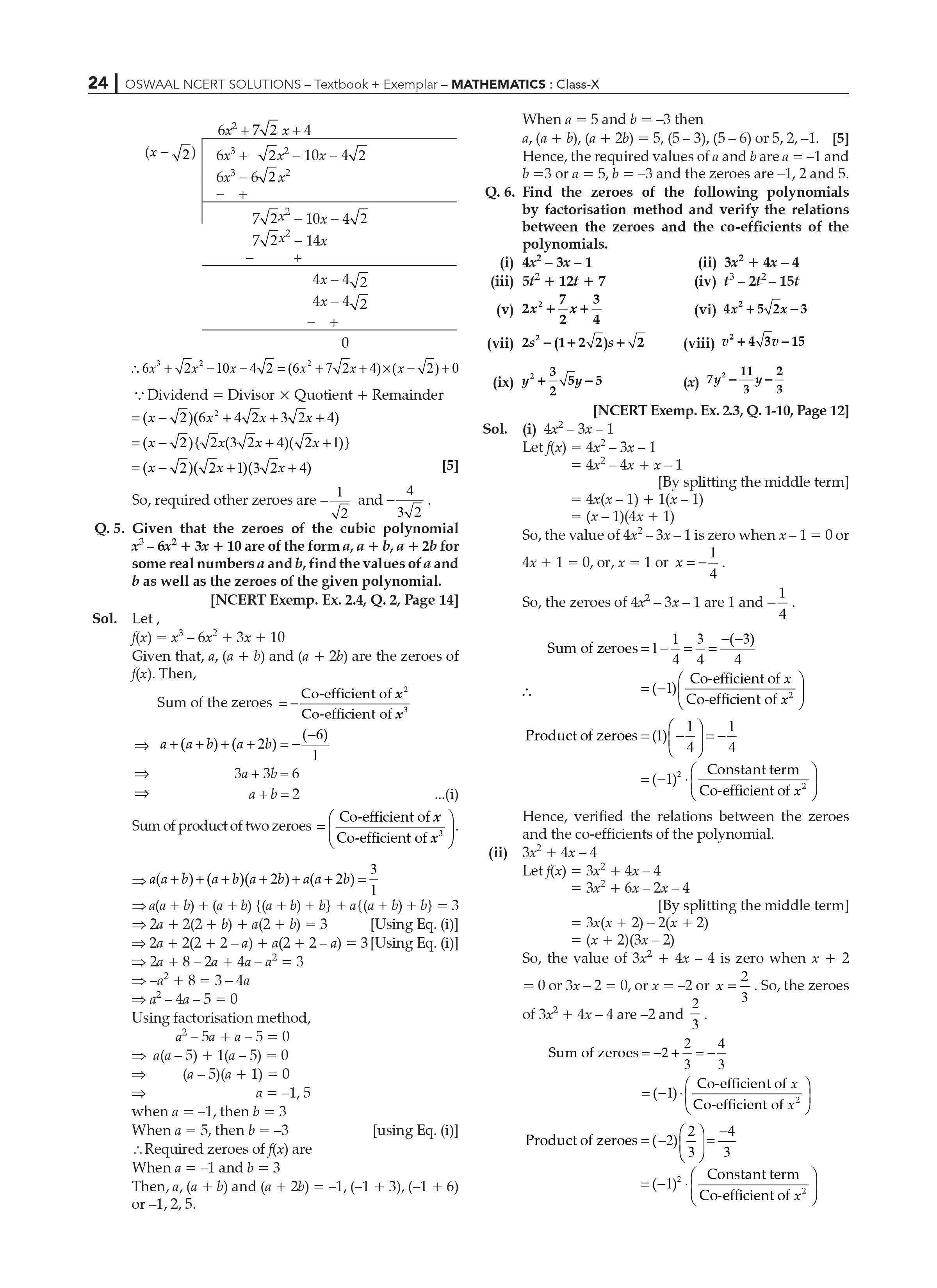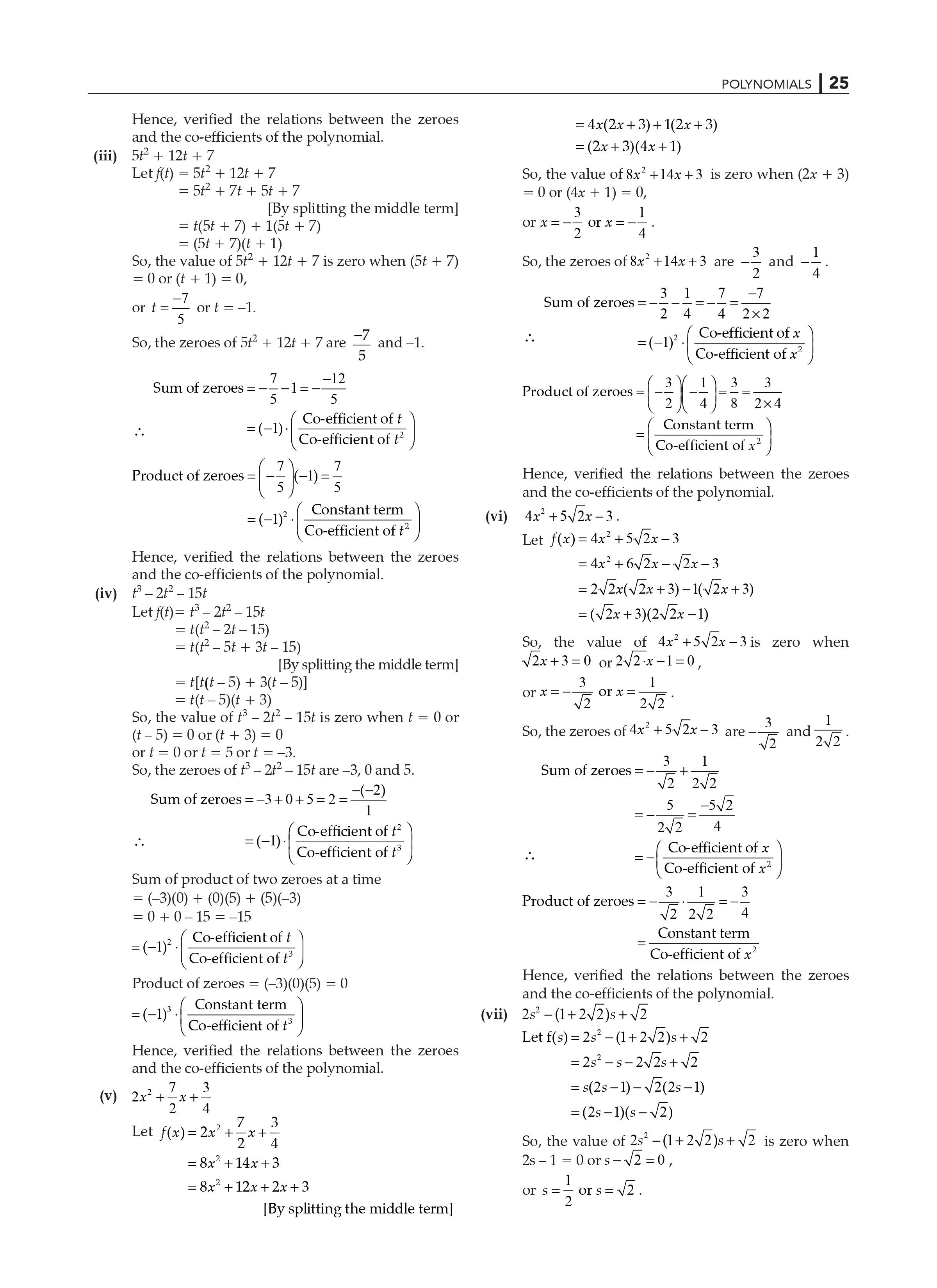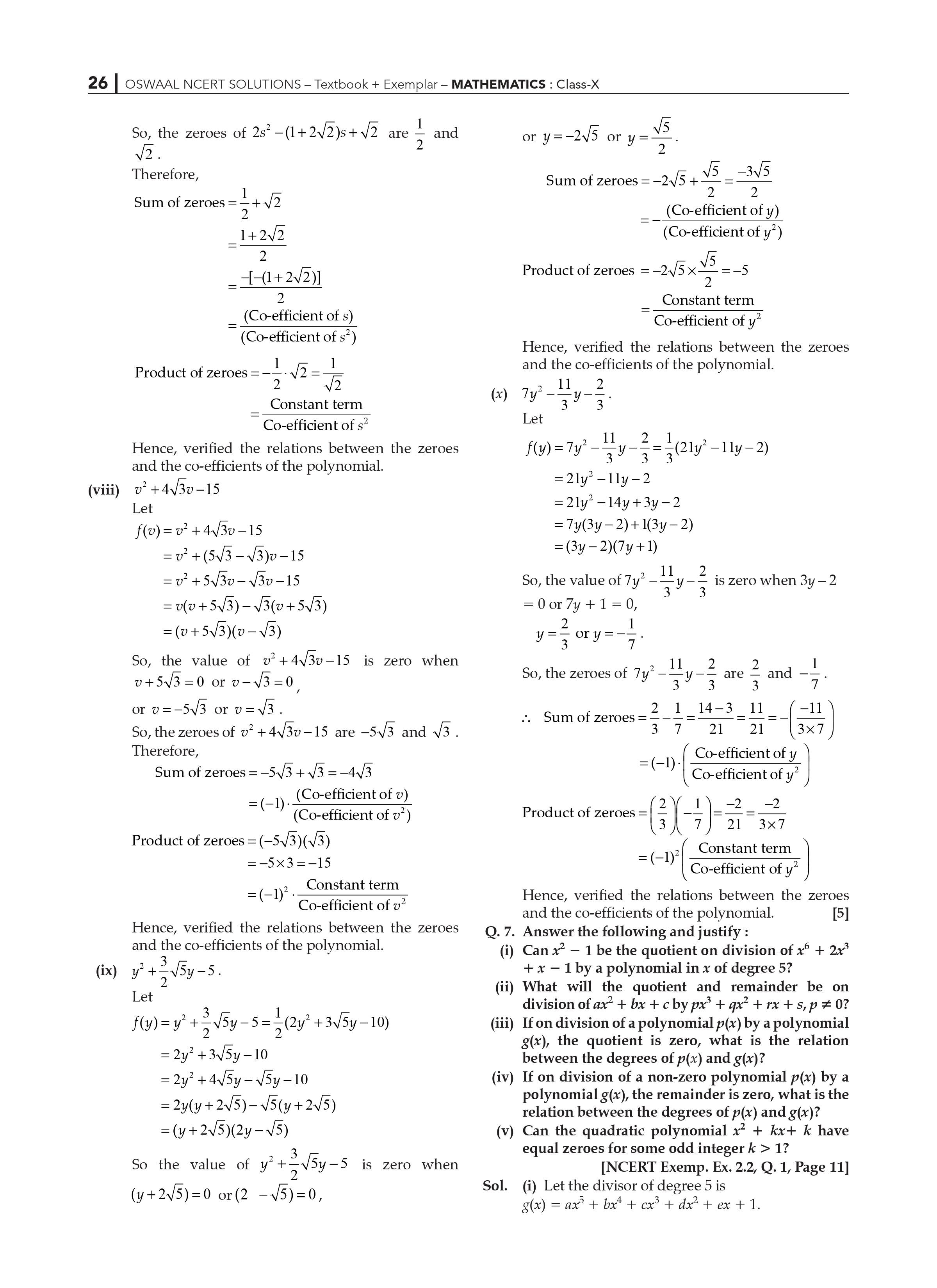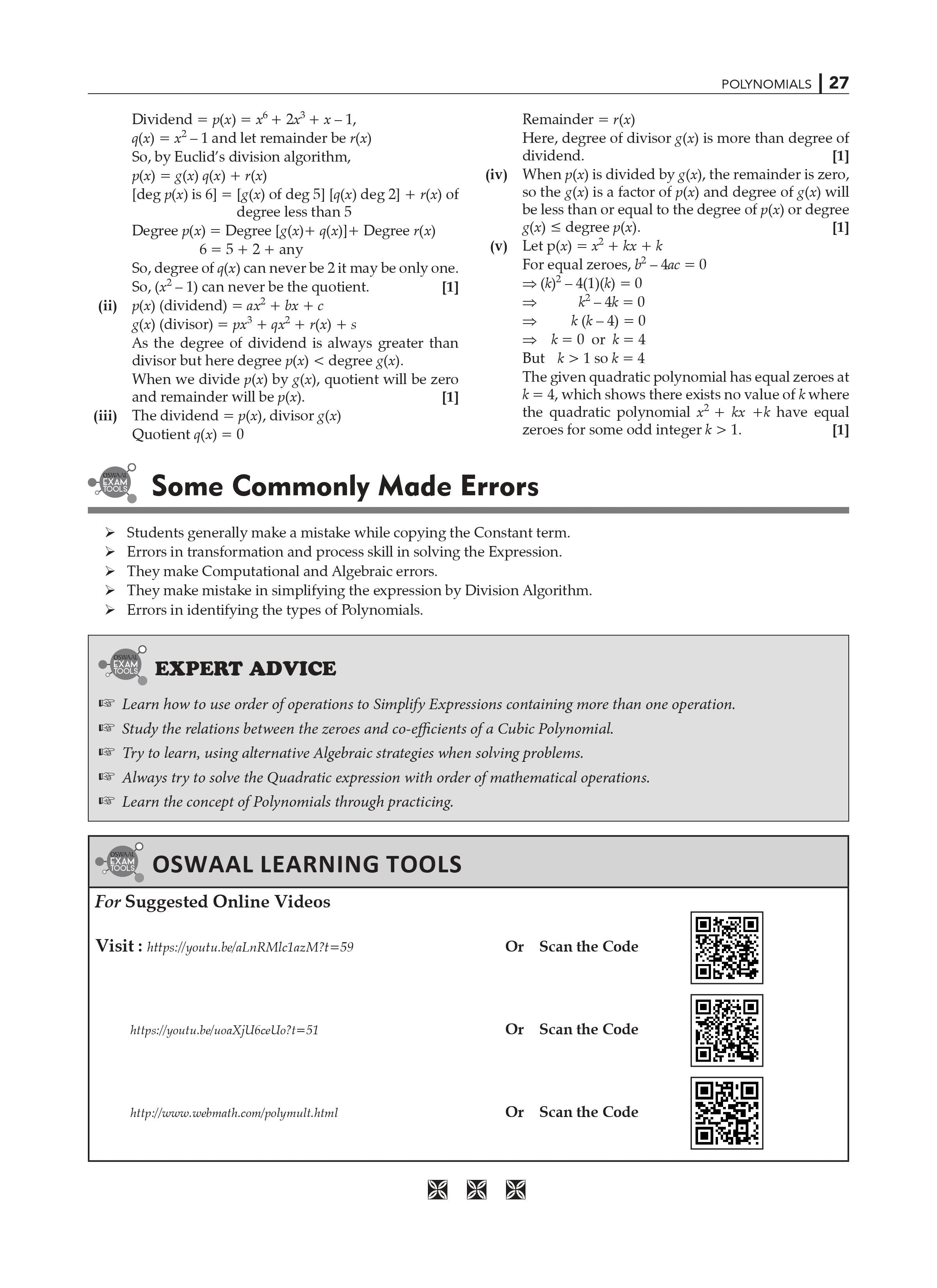This chapter discusses the following topics:

Polynomials: An Overview

Relationship between Zeros and Coefficients of a Polynomial Division Algorithm for Polynomials has a geometrical meaning.

After discussing polynomials in one variable and their degrees in the previous lesson, Lesson 10 delves deeper into polynomials. The answers to a range of questions about polynomials and their applications are covered in this chapter of NCERT Solutions for Class 10 Maths. We look into the relationship between the zeros of quadratic polynomials and their coefficients, as well as the division technique for polynomials of integers.

Polynomials are introduced in section 2.1 of the chapter, followed by parts 2.2 and 2.3, which address two extremely important topics.

Geometrical Meaning of a Polynomial's Zeroes - There is one question with six different instances.

Relationship between a polynomial's zeros and coefficients – Examine the relationship between a quadratic polynomial's zeroes and coefficients by solving two problems in Exercise 2.2, each of which has six parts.

NCERT Solutions for Class 10 Maths Chapter 2: Polynomials: Key Features:

• It covers the new CBSE Class 10 Maths term-by-term syllabus for 2021-22.
• You will be confident in your ability to achieve well in exams after studying these NCERT Solutions prepared by our subject specialists.
• It follows NCERT guidelines, which aid in students' thorough preparation.
• It contains all of the important questions from the standpoint of the examination.

Here are the notes for CBSE Class 10 Maths Chapter 2 Polynomial. With several examples, we will cover everything from what is a polynomial and its kinds to algebraic expressions, degree of a polynomial expression, graphical representation of polynomial equations, factorization, and the link between zeroes and the coefficient of a polynomial.

Algebraic Expression

An algebraic expression is a formula that contains variables, constants, and mathematical operators.

A sum of terms, often known as building blocks for expressions, is an algebraic expression.

Variables and constants are combined to form a term. In some cases, a term can be an algebraic expression in and of itself.

- 3 is an example of a phrase that is simply a constant.

- 2x is the product of the constant '2' and the variable 'x'.

- 4xy is the product of the constant '4' and the variables 'x' and 'y'.

- 5x2y, which is the sum of the numbers 5, x, x, and y.

The coefficient refers to the constant in each expression.

3x2y+4xy+5x+6 is an algebraic expression that is the sum of four terms: 3x2y, 4xy, 5xy, and 6.

Polynomial

An algebraic expression is a formula that contains variables, constants, and mathematical operators.

Exponents in algebraic expressions can be rational values. A polynomial, on the other hand, is an algebraic expression in which the exponent on any variable is a whole integer; an example of a polynomial is 5x3+3x+1. It's also an algebraic expression.

2x+3x is a polynomial, however it is not an algebraic expression. because x has an exponent of 1/2, which is not a whole number.

Degree of a Polynomial

The degree of a polynomial in one variable is equal to the largest exponent on the variable in the polynomial.

Example: The polynomial x2+2x+3 has a degree of 2 since the largest power of x in the provided expression is x2.

Polynomials classified by the number of terms

A one term polynomial is monomial. 2x, 6x2, 9xy, etc.

Binomial – A two-term polynomial. 4x2+x, 5x+4 are two examples.

A trinomial is a polynomial with three terms. x2+3x+4 is an example.

Degree Polynomials Types: -

A linear polynomial is a polynomial that has only one degree.

A linear polynomial, for example, is 2x+1.

Quadratic Polynomial is a type of polynomial that has four coefficients

A quadratic polynomial is a polynomial with two degrees.

3x2+8x+5 is a quadratic polynomial, for example.

 Extra Information: NCERT Exemplar for Class 10 Maths Chapter 2 CBSE Notes for Class 10 Maths Chapter 2

NCERT Solutions for class 10 Maths Chapter 2- Polynomials Exercise 2.1

NCERT Solutions of Chapter 2 for Class 10 Maths have been solved here with detailed explanation by our specialists of the particular subject. These solutions are students’ friendly solutions as these are directly linked from the concepts students last studied in the previous grade.

All the NCERT guidelines are considered strongly by our specialists while preparing solutions. The solutions are checked by our professionals after our specialists complete them so as to make the solutions error free. These solutions work on building the concepts of students so that they never get any confusion in these particular topics.

Exercise 2.1 Polynomials Class 10 Maths, covers the concept of zeroes of a polynomial. This section also includes the geometrical representation and meaning of linear and quadratic polynomials.

One liner explanation of the concept’s students will learn in this particular of NCERT chapter 2.

Zeroes of a polynomials may be defined as the points on which the value of polynomial becomes zero; for example, let us assume a polynomial p(x) and at any point k value of p(x) is zero, i.e., p(k)=0. Thus, k is the zero of polynomial p(x). Geometrically, we can say that at point k, the polynomial will cut the x- axis.

Chapter 2 - Polynomials NCERT Solutions for Class 10 Maths:

The first exercise in Chapter 2 of Class 10 Maths is Exercise 2.1 which continues from where we left the concept of polynomials in Class 9. Polynomials are introduced in Class 9 and examined in further depth in Class 10 by looking at various situations of the geometrical significance of a polynomial's zeroes.

Key Benefits of NCERT Solutions for Class 10 Maths Chapter 2- Polynomials Exercise 2.1:

• You can use these NCERT Solutions to solve and revise NCERT exercise 2.1 questions.
• It will build your concepts as well as it will boost up your confidence which you need, to take the Class 10 CBSE 2023 exams.
• It explains the concept of polynomials in the detailed manner and to understand better it is important to solve each question given in the NCERT textbook exercises of the chapter.
• It is very important to understand the idea of polynomials and their zeroes with the use of graphs. Zeroes of the polynomials, also known as roots of the polynomials are very essential as these are the solutions for any given polynomial for which the unknown variable's value must be determined. If we know the roots, we can evaluate the value of a polynomial to zero.

Frequently Asked Questions on NCERT Solutions of Chapter 2 Exercise 2.1:

What is the weightage of this Chapter 2 Exercise 2.1 in CBSE examination?

Answer: Chapter 2 Exercise 2.1 of Class 10 Maths is very important from examination point of view as well. It will constitute approximately 4 marks of the total marks of the exam paper.

Is it important to solve NCERT solutions?

Answer: Yes, it is very important to solve NCERT questions as it clears the concepts of the questions which will come in very handy even if the examiner twists the language or the pattern of the basic questions.

What is the importance of Chapter 2 Exercise 2.1 of Class 10?

Answer: Chapter 2 Exercise 2.1 of Class 10 is considered as the most significant chapter in Class 10 Math because you will gain a proper understanding of the polynomial equations which will help you in later chapters. Understanding the graph and connecting it to the equation is fundamental as you will be asked similar questions in subsequent chapters. As a result, try to attempt answer as many questions as possible in order to gain a thorough comprehension of the subject.

NCERT Solutions for class 10 Maths Chapter 2- Polynomials Exercise 2.2

NCERT Solutions for Class 10 Maths, Chapter 2 Exercise 2.2, have been provided here with full explanations by our subject experts. These are student-friendly solutions since they are directly related to subjects that students previously covered in the preceding grade. When preparing solutions, our experts take into account all of the NCERT requirements.

Our professionals evaluate the solutions once our specialists finish them to ensure that they are error-free. These solutions help students to develop their notions so that they are never confused about these concepts. Students can use these NCERT chapter-by-chapter solutions to study and prepare for their CBSE 2023 exams. NCERT Exercise 2.2 Chapter 2- polynomials Solutions are presented in a step-by-step and easy-to-understand manner. Once you've gone over these NCERT solutions for Class 10 Maths, you'll be able to solve them quickly.

NCERT Exercise 2.2 Chapter 2- polynomials, illustrate the relationship between the zeroes of a polynomial with the coefficient of polynomials.

Brief explanation of exercise 2.2 class 10 Maths: The relationship of zeroes of polynomial with coefficients of polynomials. In this exercise Linear polynomial and quadratic polynomials are discussed. We already know that zeroes of a linear polynomial can be calculated by simply putting the linear polynomial, p(x)= ax+b equal to zero and find the value of x which will turn out to be -b/a.

Now, quadratic polynomial having degree 2 and it will relate the sum of the two roots and product of the two roots with the coefficient of quadratic polynomial.

The two roots are represented by two Greek symbols α and β.

Chapter 2 - Polynomials NCERT Solutions for Class 10 Maths:  The second exercise in Chapter 2 of Class 10 Maths is Exercise 2.2 solely explains how to find sum of roots of a polynomial and product of roots of polynomial without actually finding the roots of the equation. It is done by using the relationship between the sum of roots and the product of roots with the coefficient of polynomials respectively.

NCERT Solutions for Class 10 Maths Chapter 2- Polynomials: Key Benefits

• These NCERT Solutions will assist you in answering and revising all of the problems in Exercise 2.1.
• It adheres to NCERT criteria, which aid in the proper preparation of students.
• From the standpoint of the examination, it comprises all of the key questions and solving these NCERT questions will help you to do better in the examination.

1. Is it important to solve NCERT solutions?

Answer: Yes, solving NCERT questions is very significant since it clarifies the principles of the questions, which will come in helpful even if the examiner twists the language or pattern of the core questions.

1. What is the weightage of this Chapter 2 in CBSE examination?

Answer: Chapter 2 of Class 10 Maths is also highly crucial in terms of examinations. It will account for about 4 percent of the total marks on the exam paper.

1. Is it important to solve examples before the exercise question?

Answer: Yes, it is important to solve the examples as well as these are the basis of the concepts as well as the questions in the further exercise. NCERT examples are designed to assist students in a timely manner in the event of questions or uncertainties. The answers are thoroughly presented, with examples that are both relevant and understandable.

1. What is the summary of exercise 2.2 Chapter 2 polynomials?

Answer: This exercise states that the sum of zeroes of an equation is equal to - (coefficient of x) divided by the coefficient of square of x. The zero product is equal to the constant term divided by the coefficient of square of x. The concept is taught using examples and the practice questions from the textbook.

NCERT Solutions for Class 10 Maths Chapter 2- Polynomials Exercise 2.3

NCERT Solutions for 10 Maths Exercise 2.3 Chapter 2: Our topic specialists have ready the polynomials that you're going to notice here. These solutions area unit written by NCERT topic specialists so evaluated by them to make sure that students perceive the thought. This guarantees that the NCERT Solutions area unit easy enough for students of CBSE class 10 to grasp.

In Chapter two Polynomials class 10 Maths NCERT Exercise 2.3, The Division algorithmic rule for polynomials is mentioned. These answers area unit written with the aim of jutting to all or any NCERT rules. It conjointly makes bound that the complete topic is discussed while making an attempt to unravel issues, guaranteeing that the learner doesn't miss any topics.

1. What's the Division algorithmic rule for polynomials?

Division is also a computation throughout that things unit of measurement divided into equal elements. it's conjointly referred to as the multiplication operation's inverse.

For example, at intervals the multiplication, suppose that their unit of measurement a combine of groups of vi that make the twelve and presently if we have a tendency to tend to divide the twelve into a combine of groups there will be vi objects in each cluster. Here the twelve is presently the dividend, a combine of is that the divisor and vi is that the quotient. The Division algorithm for polynomials tells us that, if p(x) and g(x) unit of measurement the two polynomials, where g(x)≠0, we are going to write the division of polynomials as:

p(x) = q(x) * g(x) + r(x)

In this equation the p(x) denotes the dividend, q(x) denotes the quotient, g(x) denotes the divisor, r(x) denotes the rest.

Basics of Division

A dividend is also a full vary or the number of one thing that possesses to be split into equal components. Addition, subtraction, multiplication, and division unit of measurement the four basic operations on numbers. The division of variety into equal components is also a technique that leaves a remainder if the provided variety cannot be divided equally into components. thus, the division with a remainder contains the followings

* Dividend - Dividend is that the variety that's to be divided by the divisor.

* Divisor - the amount by that the dividend is to be divided is termed the divisor.

* Quotient - The resultant of the division is termed the quotient.

* Remainder - the amount that's left once division is termed the rest.

Polynomial division includes dividing one polynomial by a monomial, binomial, trinomial, or lower degree polynomial. The dividend's degree is larger than the divisor in associate passing polynomial division. to look at the result, multiply the divisor and quotient, then add the remaining, if any, to the result.

To divide a polynomial by another polynomial, follow these steps.

Step 1: organize the dividend and also the divisor so as of decreasing degrees.

Step 2: Divide the best degree term of the dividend by the best degree term of the divisor to induce the primary term of the quotient.

Step 3: Divide the best degree term of the new dividend made in step two by the biggest degree term of the divisor to induce the second term of the quotient.

Step 4: Repeat the steps once more till the degree of the rest is a smaller amount than the degree of the divisor.

To execute polynomial division, we'll use the division algorithmic rule stages.

Step one: The polynomials area unit already organized within the dropping order of their degrees.

Step 2: the primary term of the quotient is obtained by dividing the biggest degree term of the dividend with the biggest degree term of the divisor. initial Term = 2(x)*3/x=2(x)*2

Step 3: The new dividend is (x)*2+4(x)

Step 4: The second term of the quotient is obtained by dividing the biggest degree term of the new dividend obtained in step two with the biggest degree term of the divisor. Second Term = (x)*2/x=x

Step 5: The new dividend is 3(x)+3

Step 6: The third term of the quotient is obtained by dividing the biggest degree term of the new dividend obtained in step four with the biggest degree term of the divisor. Third Term = 3(x)/x=3

Step 7: The quotient is 2(x)*2+2(x)+3

p(x) = 2(x)*3+3(x)*2+4(x)+3 is that the dividend

q(x) = 2(x)*2+x+3 is that the quotient

g(x) = x+1 is that the divisor

r(x) = zero is that the remainder

NCERT Solutions for Class 10 Maths Chapter 2- Polynomials– Key Features:

These NCERT Solutions can assist you in resolution and rewriting all of the queries during this specific exercise 2.3, permitting you to possess a deeper understanding of the concept.

1. Once going over the gradual answers provided by our topic specialists, you may be able to get high grades.
2. It aids in achieving smart results on the Maths tests.
3. It adheres to NCERT criteria, that aid within the correct preparation of pupils.
4. From the stance of the check, NCERT solutions Class 10 contains all of the key queries.

NCERT Solutions for Class 10 Maths Chapter 2- Polynomials Exercise 2.4

This Chapter 2 Polynomials NCERT Solutions for Class 10 Math Students can use Exercise 2.4 to help them with their academics. These solutions were created by our Maths subject expert to help you prepare for the Class 10 2023 exams. These specialists create NCERT Math Solutions to make it easier for students to tackle NCERT questions quickly. They also consider how easy it is for you to grasp the topic and how quickly they can learn from it.

This 2.4 Math Exercise is optional, and it is not provided for the final examination point of view but it is important for you to understand many significant concepts later in Maths.

This NCERT Maths Solution for Class 10, comprises additional chapter-related questions to practice. Each response to the questions is given a full solution by our specialists. It also covers the whole syllabus of NCERT Class 10th Maths book. These will help you to score high in exams and it will also help to revise the NCERT complete syllabus.

Main Advantages of NCERT Solutions for Class 10 Maths Chapter 2- Polynomials Exercise 2.4:

• Students will be able to obtain higher grades by practicing step-by-step these NCERT solutions provided by our subject specialist teachers.
• It completely contains NCERT criteria, which benefit the students in complete preparation for exams.
• It covers the whole syllabus and also consists of all of the key questions.
• It will help the students to score well in the first-term Math examinations.
• These NCERT Solutions can also be used to solve and review all of the problems in exercise 2.4.

Why Choose Us?

We make Mathematics interesting for even those students who are scared even by the name of this subject. Highly skilled and qualified teachers have created the subject of this solution. These solutions are the best if you want to get your concepts cleared easily at the primary stage.

You don’t need to go to Maths tuition every day, all you need to do is practice these NCERT Solution papers well and it will help you to score high in the examination.

If you go through these NCERT Solution papers and practice Maths religiously, you will get to see the difference in yourself in your next examination. You don’t need to practice Maths for hours anymore. All you need to do is to go through these NCERT Maths Class 10 questions and solutions provided here. There is no important question on the Maths Chapter 2 Class 10 that we have missed in these notes.

With these NCERT Solutions for Class 10 Math, you will also learn how to solve a problem related to the Polynomial Chapter in the easiest manner possible. Once you learn it, no one can stop you from getting high marks in Maths.

This time with these notes, you will be surely able to surprise your teachers as well as your parents. Study the Exercise 2.4 Class 10 Maths NCERT Solutions for free to score better in your exams.

1. What is the best way to score full marks in NCERT Exercise 2.4 of Chapter 2 of Class 10 Maths?

Students of Class 10 can score full marks in Exercise 2.4 of Chapter 2 by practicing all questions given in the Exercise. The solutions are prepared by expert subject teachers for helping the students in concept understanding and scoring maximum marks.

1. What does NCERT Solutions Class 10th Maths Chapter 2 Exercise 2.4 deal with?

These NCERT Solutions for Class 10 Maths Chapter 2, Exercise 2.4 will help you in understanding the concept of Polynomials in a clear manner. This chapter helps students to understand many other significant concepts in Maths.

1. Studying from NCERT Solutions for Exercise 2.4 of Chapter 2 of Class 10 Maths is sufficient to prepare for the exams?

NCERT Solutions for Chapter 2 of Class 10 Maths are sufficient for the students to prepare for the exams as they can solve all NCERT solutions by practicing these solutions daily. The solutions are prepared by expert teachers by keeping this point in mind that students can score high in the examinations. Students can easily understand the pattern of the questions asked in the examinations and this can help them to solve all questions easily in the exams in a limited given time.

Frequently Asked Questions: NCERT Solutions for Class 10 Maths Chapter 2:

Where can I find the proper NCERT Solutions for Chapter 2 of Class 10 Maths?

The NCERT Textbook Solutions for Polynomials have been meticulously constructed. All of these solutions are based on the new CBSE pattern, ensuring that students comprehend the topic thoroughly in preparation for their exams.

Is it compulsory to finish all of the problems in NCERT Solutions for Class 10 Maths Chapter 2?

Yes. Because they are the most important questions in the exam. These questions have been answered by experts to help students complete the work quickly. These solutions help students have a better understanding of polynomials.

What topics are included in the NCERT Solutions for Class 10 Maths Polynomials?

The concepts are covered in the NCERT Solutions for Class 10 Maths. Polynomial division algorithm, geometrical meaning of polynomial zeros, relationship between zeros and coefficients of a polynomial, and introduction to polynomials If students are able to answer polynomial problems, they will be successful.